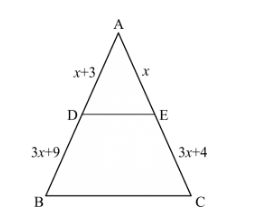# In the given figure, the value of x for which DE || AB is

Question:

In the given figure, the value of x for which DE || AB is(a) 4
(b) 1
(c) 3
(d) 2

Solution:

Given: In ∆ABC,  DE || AB.

To find: the value of x

According to basic proportionality theorem if a line is drawn parallel to one side of a triangle intersecting the other two sides, then it divides the two sides in the same ratio.

In ∆ABC,  DE || AB

$\frac{\mathrm{AD}}{\mathrm{DB}}=\frac{\mathrm{AE}}{\mathrm{EC}}$

$\frac{x+3}{3 x+19}=\frac{x}{3 x+4}$

$(x+3)(3 x+4)=(x)(3 x+19)$

$3 x^{2}+4 x+9 x+12=3 x^{2}+19 x$

$19 x-13 x=12$

$6 x=12$

$x=2$

Hence we got the result $(d)$.